Next: Spontaneous Emission of Radiation Up: Time-Dependent Perturbation Theory Previous: Harmonic Perturbations

# Absorption and Stimulated Emission of Radiation

Let us employ time-dependent perturbation theory to investigate the interaction of an electron in a hydrogen-like atom with classical (i.e., non-quantized) electromagnetic radiation. The Hamiltonian of such an electron is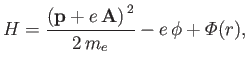(8.122)

where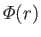is the atomic potential energy, and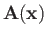and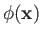are the vector and scalar potentials associated with the incident radiation. (See Section 3.6.) The previous equation can be written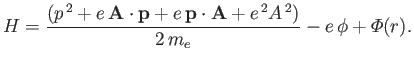(8.123)

Now,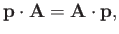(8.124)

provided that we adopt the Coulomb gauge,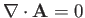. (See Exercise 6.) Hence,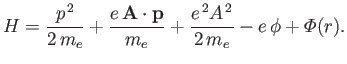(8.125)

Suppose that the perturbation corresponds to a monochromatic plane-wave of angular frequency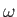, for which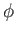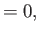(8.126)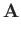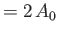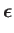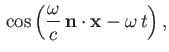(8.127)

where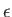and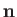are unit vectors that specify the wave's electric polarization direction (i.e., the direction of its electric component) and its direction of propagation, respectively. [The wavevector is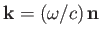.] Here,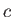is the velocity of light in vacuum. Note that, according to standard electromagnetic theory,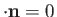. The Hamiltonian becomes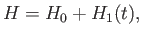(8.128)

with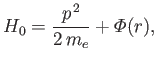(8.129)

and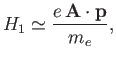(8.130)

where the term involving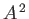, which is second order in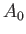, has been neglected.

The perturbing Hamiltonian can be written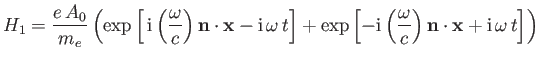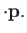(8.131)

This has the same form as Equation (8.113), provided that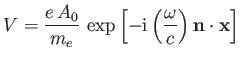(8.132)

It is clear, by analogy with the analysis of Section 8.8, that the first term on the right-hand side of Equation (8.134) describes a process by which the atom absorbs energy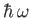from the electromagnetic field. On the other hand, the second term describes a process by which the atom emits energyto the electromagnetic field. We can interpret the former and latter processes as the absorption and stimulated emission, respectively, of a photon of energyby the atom.

It is convenient to define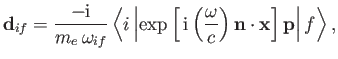(8.133)

which has the dimensions of length. Note that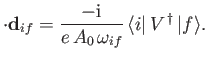(8.134)

It follows, from Equations (8.122) and (8.123), that the rates of absorption and stimulated emission are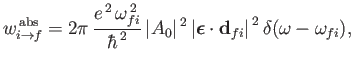(8.135)

and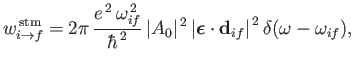(8.136)

respectively. (See Exercise 19.) It can be seen that absorption involves the absorption by the atom of a photon of angular frequency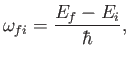(8.137)

and energy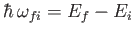, causing a transition from an atomic state with an initial energy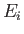to a state with a final energy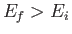. On the other hand, stimulated emission involves the emission by the atom of a photon of angular frequency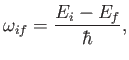(8.138)

and energy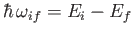, causing a transition from an atomic state with an initial energyto a state with a final energy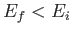. In both cases,andspecify the direction of propagation and electric polarization direction, respectively, of the photon. For the case of stimulated emission,andalso specify the direction of propagation and polarization direction, respectively, of the radiation that stimulates the atomic transition.

Now, the energy density of an electromagnetic radiation field is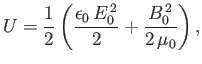(8.139)

where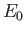and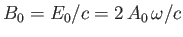are the peak electric and magnetic field-strengths, respectively. Hence,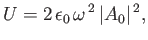(8.140)

and expressions (8.138) and (8.139) become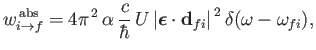(8.141)

and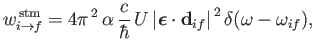(8.142)

respectively, where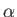is the fine structure constant.

Let us suppose that the incident radiation has a range of different angular frequencies, so that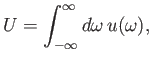(8.143)

where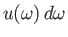is the energy density of radiation whose angular frequency lies in the rangeto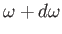. Equations (8.144) and (8.145) imply that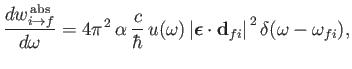(8.144)

and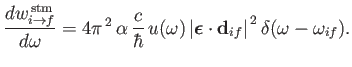(8.145)

Here,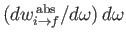is the rate of absorption associated with radiation whose angular frequency lies in the rangeto, et cetera. Incidentally, we are assuming that the radiation is incoherent, so that intensities can be added . Of course,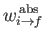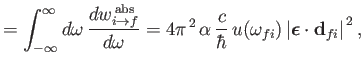(8.146)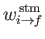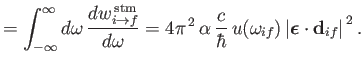(8.147)

The rate at which the atom gains energy from the electromagnetic field as a consequence of absorption can be written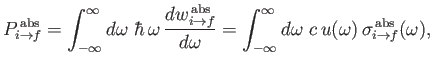(8.148)

where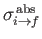is the so-called absorption cross-section, and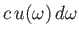the electromagnetic energy flux associated with radiation whose angular frequency lies in the rangeto. It follows that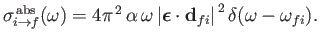(8.149)

Similarly, the stimulated emission cross-section takes the form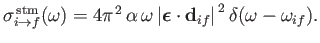(8.150)

Finally, comparing Equations (8.149) and (8.150) with the previous two equations, we deduce that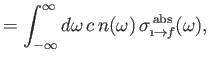(8.151)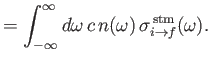(8.152)

Here, we have written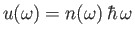, where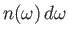is the number density of photons whose angular frequencies lie in the rangeto.Next: Spontaneous Emission of Radiation Up: Time-Dependent Perturbation Theory Previous: Harmonic Perturbations
Richard Fitzpatrick 2016-01-22